## Algebra

### Simplifying Rational Expressions

Given a rational expression, the quotient of two polynomials, we will factor the numerator and denominator if we can and then cancel factors that are exactly the same.When evaluating rational expressions, plug in the appropriate values either before simplifying or after, the result will be the same.  Although, it is more efficient to simplify first then evaluate.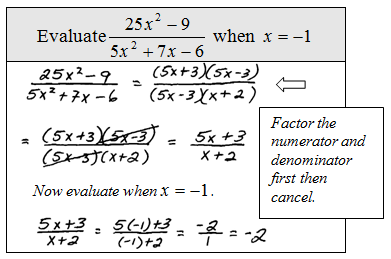We can see that when evaluating, the result will be the same whether or not we simplify first.  It turns out that not all numbers can be used when we evaluate.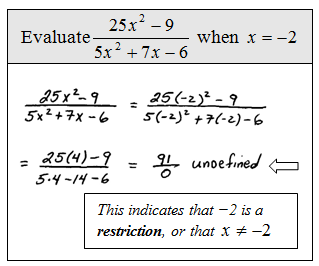The point is that not all real numbers will be defined in the above rational expression.  In fact, there are two restrictions to the domain, -2 and 3/5.  These values, when plugged in, will result in zero in the denominator.  Another way to say this is that the domain consists of all real numbers except for −2 and 3/5.

Tip: To find the restrictions, set each factor in the denominator equal to zero and solve. The factors in the numerator do not contribute to the list of restrictions.

Simplify and state the restrictions to the domain.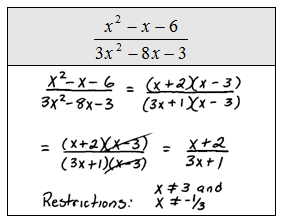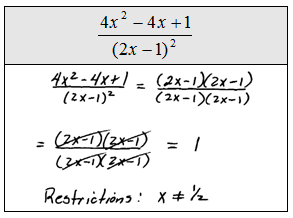Even if the factor cancels it still contributes to the list of restrictions.  Basically, it is important to remember the domain of the original expression when simplifying. Also, we must use caution when simplifying, please do not try to take obviously incorrect shortcuts like this: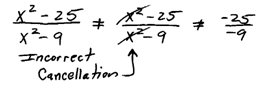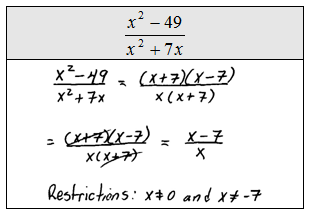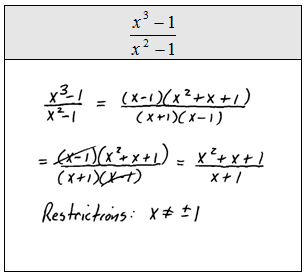Since subtraction is not commutative, we must be alert to opposite binomial factors.  For example, 5 − 3 = 2 and 3 − 5 = −2. In general,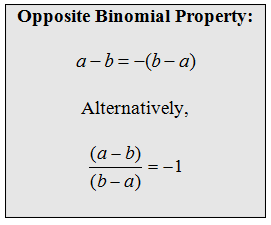Simplify and state the restrictions to the domain.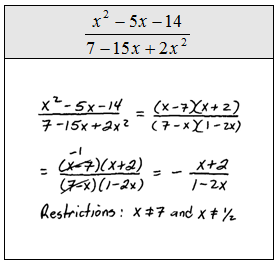At this point, we evaluate using function notation.